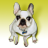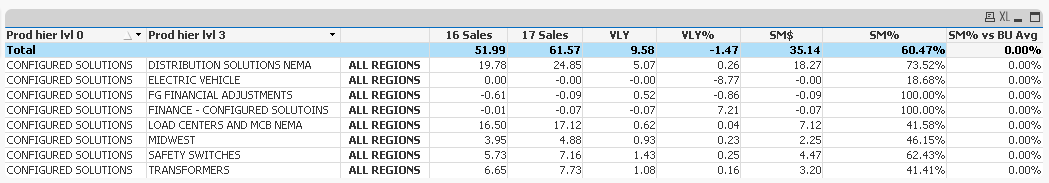cancel
Showing results for
Did you mean:Contributor III

## Subtracting row value from total column avg in straight table

Hi, In the last column I am trying to populate values from SM% Column by subtracting the row value minus total avg i.e. 73.52-60.47 =, like wise for rest of the rows. can someone please help me on this.

SM% = SM\$/Column(2)

** I have selected one Prod hier lvl0 here but we have similarly having 5 values under this lvl 0. Under each lvl 0 we have few lvl 3 values as shown here. so the calculation is driven by lvl0 & lvl3 & Pole. lvl 3s is under lvl0.13 RepliesMVP

Can you please share whole expression rather Column() and Label names of expression, please?

Before develop something, think If placed (The Right information | To the right people | At the Right time | In the Right place | With the Right context)Contributor III
Author

Column(2) => IF(POLE_ILT = 'ALL REGIONS',

Sum({< Transaction_Type = {'Billings'}, Year_Fiscal = {\$(#vFYear)}, Quarter_Fiscal = {\$(#vFQuarter)}, POLE = >} USD_NET_SALES)/1000000)

SM\$ => IF(POLE_ILT = 'ALL REGIONS', Sum({< Transaction_Type = {'Billings'}, Year_Fiscal = {\$(#vFYear)},Quarter_Fiscal = {\$(#vFQuarter)}, POLE = >} USD_NET_SALES_MARGIN)/1000000)

SM% => if(Column(2)=0,0, (SM\$/Column(2)))MVP

This is really intensive and i am aggressive to sort out. Perhaps this?

if(Column(2)=0,0, (SM\$/Column(2)))

If((Sum({< Transaction_Type = {'Billings'}, Year_Fiscal = {\$(#vFYear)},Quarter_Fiscal = {\$(#vFQuarter)}, POLE = , POLE_ILT = {'ALL REGIONS'}>} USD_NET_SALES_MARGIN)/1000000)) = 0, 0,

(Sum({< Transaction_Type = {'Billings'}, Year_Fiscal = {\$(#vFYear)}, Quarter_Fiscal = {\$(#vFQuarter)}, POLE = , POLE_ILT = {'ALL REGIONS'}>} USD_NET_SALES)/1000000))/

(Sum({< Transaction_Type = {'Billings'}, Year_Fiscal = {\$(#vFYear)},Quarter_Fiscal = {\$(#vFQuarter)}, POLE = , POLE_ILT = {'ALL REGIONS'}>} USD_NET_SALES_MARGIN)/1000000)))

-

if(Column(2)=0,0, (SM\$/Column(2)))

If((Sum({< Transaction_Type = {'Billings'}, Year_Fiscal = {\$(#vFYear)},Quarter_Fiscal = {\$(#vFQuarter)}, POLE = , POLE_ILT = {'ALL REGIONS'}>} TOTAL USD_NET_SALES_MARGIN)/1000000)) = 0, 0,

(Sum({< Transaction_Type = {'Billings'}, Year_Fiscal = {\$(#vFYear)}, Quarter_Fiscal = {\$(#vFQuarter)}, POLE = , POLE_ILT = {'ALL REGIONS'}>} TOTAL USD_NET_SALES)/1000000))/

(Sum({< Transaction_Type = {'Billings'}, Year_Fiscal = {\$(#vFYear)},Quarter_Fiscal = {\$(#vFQuarter)}, POLE = , POLE_ILT = {'ALL REGIONS'}>} TOTAL USD_NET_SALES_MARGIN)/1000000)))

Before develop something, think If placed (The Right information | To the right people | At the Right time | In the Right place | With the Right context)Contributor III
Author

Thanks Anil. But I believe you want me to use the expressions in the calculation instead of Cloumn() function. correct me if I am wrong.

Also what I am looking for is the calculation 'SM% vs BU Avg' which is like 73.52-60.47 = x, then 18.68 - 60.47 = y etc etc.

Note: ** 60.47 is the avg value of the SM% column AND I set this in the expression tab by selecting Avg

Also to simplify the SM% formula let's use SM\$/Column(2) instead of any error handling. i.e. SM% = SM\$/Column(2)MVP

And, For that you just copy paste in your Avg column and check. Or else, Please post suitable work file to investigate.

Before develop something, think If placed (The Right information | To the right people | At the Right time | In the Right place | With the Right context)Contributor III
Author

Hi Anil, can we any way capture that total avg (60.47) or we need to calculate it? I am not able to generate the count based on lvl 0 & lvl3. I was thinking of summing up the SM% and then divide it with the count of lvl3.Contributor III
Author

Hi Anil, Please find a working copy. Do let me know if you need any more information.MVP

Can you try this using text box and tell us the values you are getting in your real application.

=Avg(Aggr(If(POLE_ILT = 'ALL REGIONS', if(

IF(POLE_ILT = 'ALL REGIONS',

Sum({< Transaction_Type = {'Billings'}, Year_Fiscal = {\$(#vFYear)}, Quarter_Fiscal = {\$(#vFQuarter)}, POLE = >} TOTAL USD_NET_SALES)/1000000)=0,0,

((IF(POLE_ILT = 'ALL REGIONS', Sum({< Transaction_Type = {'Billings'}, Year_Fiscal = {\$(#vFYear)},Quarter_Fiscal = {\$(#vFQuarter)}, POLE = >} TOTAL USD_NET_SALES_MARGIN)/1000000))/

(IF(POLE_ILT = 'ALL REGIONS',

Sum({< Transaction_Type = {'Billings'}, Year_Fiscal = {\$(#vFYear)}, Quarter_Fiscal = {\$(#vFQuarter)}, POLE = >} TOTAL USD_NET_SALES)/1000000))))), [Prod hier lvl 0], [Prod hier lvl 3],

Only({<POLE_ILT = {'ALL REGIONS'}>} POLE_ILT)))

Before develop something, think If placed (The Right information | To the right people | At the Right time | In the Right place | With the Right context)Contributor III
Author

In the test box its saying nested aggregation not allowed and in real table if i put it its giving me 0 in the total value and '-' in the row.Community Browser